Download the eSaral app and start learning from Kota's top IITians and doctors.

# Simple Harmonic Motion - JEE Main Previous Year Questions with Solutions

JEE Main Previous Year Question of Physics with Solutions are available here. Practicing JEE Main Previous Year Papers Questions of Physics will help all the JEE aspirants in realizing the question pattern as well as help in analyzing their weak & strong areas. Get detailed Class 11th &12th Physics Notes to prepare for Boards as well as competitive exams like IIT JEE, NEET etc. eSaral helps the students in clearing and understanding each topic in a better way. eSaral is providing complete chapter-wise notes of Class 11th and 12th both for all subjects. Besides this, eSaral also offers NCERT Solutions, Previous year questions for JEE Main and Advance, Practice questions, Test Series for JEE Main, JEE Advanced and NEET, Important questions of Physics, Chemistry, Math, and Biology and many more. Download eSaral app for free study material and video tutorials.   Simulator Previous Years AIEEE/JEE Mains Questions
Q. If x, v and a denote the displacement, the velocity and the acceleration of a particle executing simple harmonic motion of time period T, then, which of the following does not change with time ? $(1) \mathrm{aT}+2 \pi \mathrm{v}$ (2) $\frac{\mathrm{aT}}{\mathrm{v}}$ (3) $a^{2} \mathrm{T}^{2}+4 \pi^{2} \mathrm{v}^{2}$ (4) $\frac{\mathrm{aT}}{\mathrm{x}}$ [AIEEE-2009]
Ans. (3,4) \frac{\mathrm{aT}}{\mathrm{x}}=\frac{\omega^{2} \mathrm{xT}}{\mathrm{x}}=\frac{4 \pi^{2}}{\mathrm{T}}=\mathrm{constant} \mathrm{a}^{2} \mathrm{T}^{2}+4 \pi^{2} \mathrm{v}^{2} =\left(\omega^{2} \mathrm{x}\right)^{2} \mathrm{T}^{2}+4 \pi^{2} \omega^{2}\left(\mathrm{A}^{2}-\mathrm{x}^{2}\right) =\left(\frac{4 \pi}{\mathrm{T}^{2}}\right)^{2} \mathrm{x}^{2} \cdot \mathrm{T}^{2}+4 \pi^{2} \cdot \frac{4 \pi^{2}}{\mathrm{T}^{2}} \cdot\left(\mathrm{A}^{2}-\mathrm{x}^{2}\right) $=\frac{16 \pi^{2}}{\mathrm{T}^{2}} \mathrm{A}^{2}=$ constant
Q. A mass M, attached to a horizontal spring, executes S.H.M. with amplitude $\mathrm{A}_{1}$. When the mass M passes through its mean position then a smaller mass m is placed over it and both of them move together with amplitude $\mathrm{A}_{2}$. The ratio of $\left(\frac{\mathrm{A}_{1}}{\mathrm{A}_{2}}\right)$ is :- ( 1)$\left(\frac{\mathrm{M}}{\mathrm{M}+\mathrm{m}}\right)^{1 / 2}$ ( 2)$\left(\frac{\mathrm{M}+\mathrm{m}}{\mathrm{M}}\right)^{1 / 2}$ (3) $\frac{\mathrm{M}}{\mathrm{M}+\mathrm{m}}$ (4) $\frac{\mathrm{M}+\mathrm{m}}{\mathrm{M}}$ [AIEEE-2011]
Ans. (2) Energy of simple harmonic oscillator is constant. $\Rightarrow \frac{1}{2} \mathrm{M} \omega^{2} \mathrm{A}_{1}^{2}=\frac{1}{2}(\mathrm{m}+\mathrm{M}) \omega^{2} \mathrm{A}_{2}^{2}$ $\frac{\mathrm{A}_{1}^{2}}{\mathrm{A}_{2}^{2}}=\frac{\mathrm{M}+\mathrm{m}}{\mathrm{M}}$ $\therefore \frac{\mathrm{A}_{1}}{\mathrm{A}_{2}}=\sqrt{\frac{\mathrm{M}+\mathrm{m}}{\mathrm{M}}}$
Q. Two particles are executing simple harmonic motion of the same amplitude A and frequency  along the x-axis. Their mean position is separated by distance $\mathrm{X}_{0}\left(\mathrm{X}_{0}>\mathrm{A}\right)$. If the maximum separation between them is $\left(\mathrm{X}_{0}+\mathrm{A}\right)$, the phase difference between their motion is :- (1) $\frac{\pi}{4}$ (2) $\frac{\pi}{6}$ (3) $\frac{\pi}{2}$ (4) $\frac{\pi}{3}$ [AIEEE-2011]
Ans. (4) $\mathrm{x}_{1}=\mathrm{A} \sin \left(\omega \mathrm{t}+\phi_{1}\right)$ $\mathrm{x}_{2}=\mathrm{A} \sin \left(\omega \mathrm{t}+\phi_{2}\right)$ $\mathrm{x}_{1}-\mathrm{x}_{2}=\mathrm{A}\left[2 \sin \left[\omega \mathrm{t}+\frac{\phi_{1}+\phi_{2}}{2}\right] \sin \left[\frac{\phi_{1}-\phi_{2}}{2}\right]\right]$$\mathrm{A}=2 \mathrm{A} \sin \left(\frac{\phi_{1}-\phi_{2}}{2}\right)$ $\frac{\phi_{1}-\phi_{2}}{2}=\frac{\pi}{6}$ $\phi_{1}=\frac{\pi}{3}$
Q. If a spring of stiffness' $\mathrm{k}^{\prime}$ 'is cut into two parts' 'A' and 'B' of length $\ell_{\mathrm{A}}: \ell_{\mathrm{B}}=2: 3,$ then the stiffness of spring 'A' is given by :- (1) $\frac{5}{2} \mathrm{k}$ (2) $\frac{3 k}{5}$ (3) $\frac{2 \mathrm{k}}{5}$ (4) k [AIEEE-2011]
Ans. (1) We know that, $\mathrm{k} \propto \frac{1}{\ell_{0}}$ $\therefore \mathrm{k}_{\mathrm{A}}=\frac{5}{2} \mathrm{k}$
Q. If a simple pendulum has Significant amplitude (up to a factor of 1/ e of original) only in the period between $\mathrm{t}=0 \mathrm{s}$ to $\mathrm{t}=\tau \mathrm{s}$, then $\tau$ may be called the average life ofthe pendulum. When the spherical bob of the pendulum suffers a retardation (due to viscous drag) proportional to its velocity, with 'b' as the constant of proportionality, the average lifetime ofthe pendulum is (assuming damping is small) in seconds: (1) $\frac{1}{b}$ (2) $\frac{2}{\mathrm{b}}$ (3) $\frac{0.693}{\mathrm{b}}$ (4) b [AIEEE-2012]
Ans. (2) Average life $=\tau=\frac{2}{\mathrm{b}}$
Q. The amplitude of adamped oscillator decreases to 0.9 times its original magnitude in 5s. In another 10s it will decrease to a times its original magnitude, where a equals (1) 0.81            (2) 0.729               (3) 0.6                  (4) 0.7 [JEE Main-2013]
Ans. (2) $\mathrm{A}=\mathrm{A}_{0} \mathrm{e}^{-\mathrm{t} / \mathrm{e}} \Rightarrow \frac{\mathrm{A}_{2}}{\mathrm{A}_{1}}=\frac{\mathrm{e}^{-\mathrm{t}_{2} / \mathrm{e}}}{\mathrm{e}^{-\mathrm{t} / \mathrm{e}}}=\frac{\left(\frac{\mathrm{t}_{1}-\mathrm{t}_{2}}{\tau}\right)}{\mathrm{e}}=\frac{-10}{\mathrm{c}}$ Also $0.9=\mathrm{A}_{0} \mathrm{e}^{-5 / \mathrm{c}} \Rightarrow \mathrm{e}^{-5 / \mathrm{c}}=0.9$ so $\mathrm{A}_{2}=\left(0.9 \mathrm{A}_{0}\right)(0.9)^{2}=0.729 \mathrm{A}_{0}$
Q. A particle moves with simple harmonic motion in a straight line. In first $\tau$ s, after starting from rest it travels a distance a, and in next $\tau$ s it travels $2 a,$ in same direction, then : (1) Amplitude of motion is 4a (2) Time period of oscillation is $6 \tau$ (3) Amplitude of motion is 3a (4) Time period of oscillation is $8 \tau$ [JEE Mains-2014]
Ans. (4)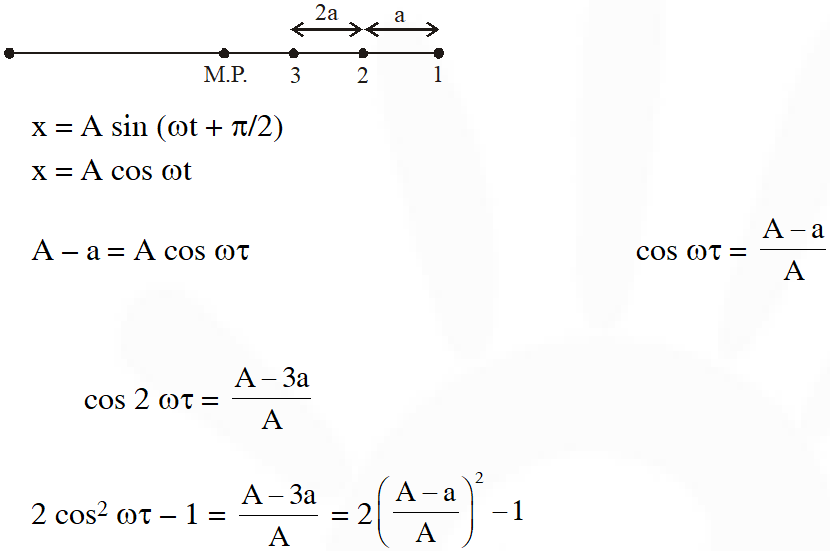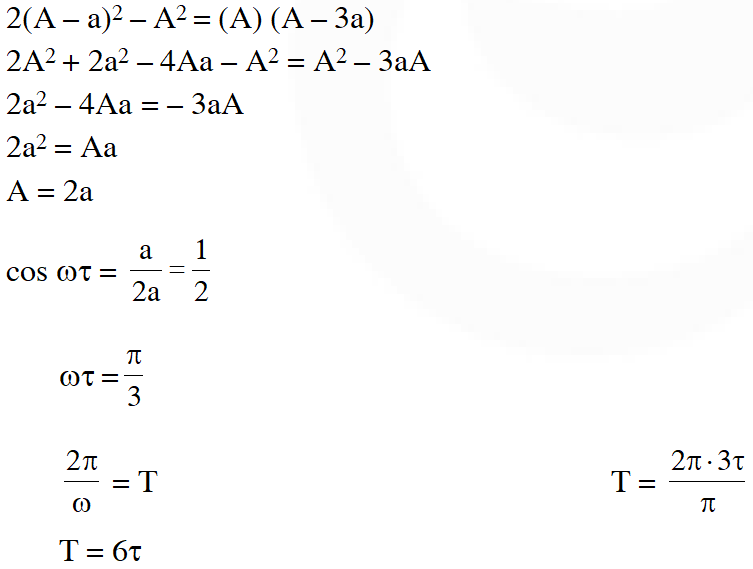Q. For a simple pendulum, a graph is plotted between its kinetic energy (KE) and potential energy (PE) against its displacement d. Which one of the following represents these correctly ? (graphs are schematic and not drawn to scale)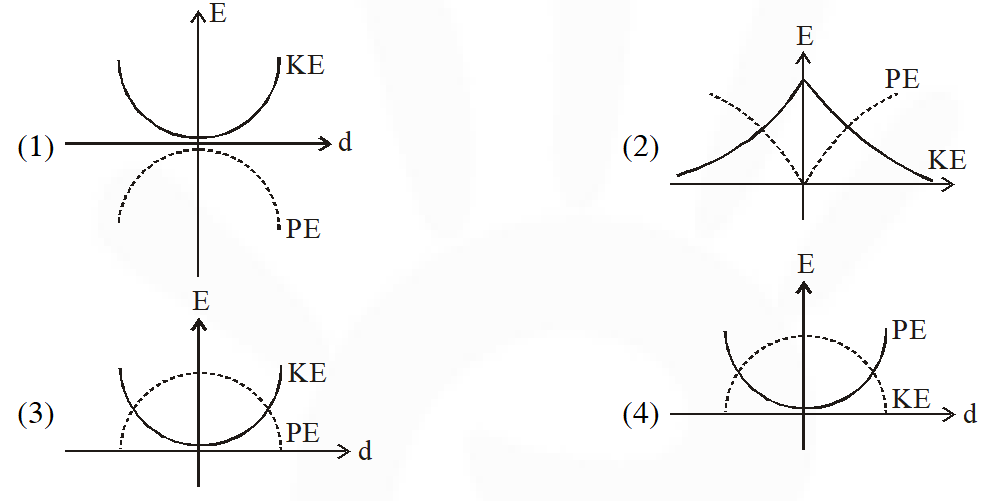[JEE Mains-2015]
Ans. (4) $\mathrm{KE}=\frac{1}{2} \mathrm{m} \omega^{2}\left[\mathrm{A}^{2}-\mathrm{d}^{2}\right],$ downward parabola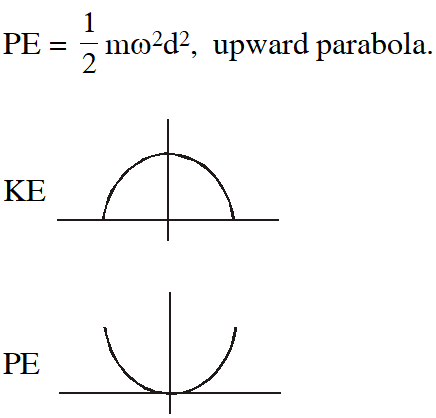Q. A particle performs simple harmonic motion with amplitude A. Its speed is trebled at the instant that it is at a distance $\frac{2 \mathrm{A}}{3}$ from equilibrium position. The new amplitude of the motion is :- (1) $\frac{7 \mathrm{A}}{3}$ (2) $\frac{\mathrm{A}}{3} \sqrt{41}$ (3) 3A (4) A $\sqrt{3}$ [JEE Mains-2016]
Ans. (1) Let new amplitude is A' initial velocity $\mathrm{v}^{2}=\omega^{2}\left(\mathrm{A}^{2}-\left(\frac{2 \mathrm{A}}{3}\right)^{2}\right) \quad \ldots(1)$ Where $\mathrm{A}$ is initial amplitude $\& \omega$ is angular frequency. Final velocity $(3 \mathrm{v})^{2}=\omega^{2}\left(\mathrm{A}^{\prime 2}-\left(\frac{2 \mathrm{A}}{3}\right)^{2}\right) \quad \ldots(2)$ From equation & equation (2) $\frac{1}{9}=\frac{A^{2}-\frac{4 A^{2}}{9}}{A^{\prime 2}-\frac{4 A^{2}}{9}}$ $\mathrm{A}^{\prime}=\frac{7 \mathrm{A}}{3}$
Q. A particle is executing simple harmonic motion with a time period T. AT time t = 0, it is at its position of equilibrium. The kinetic energy-time graph of the particle will look like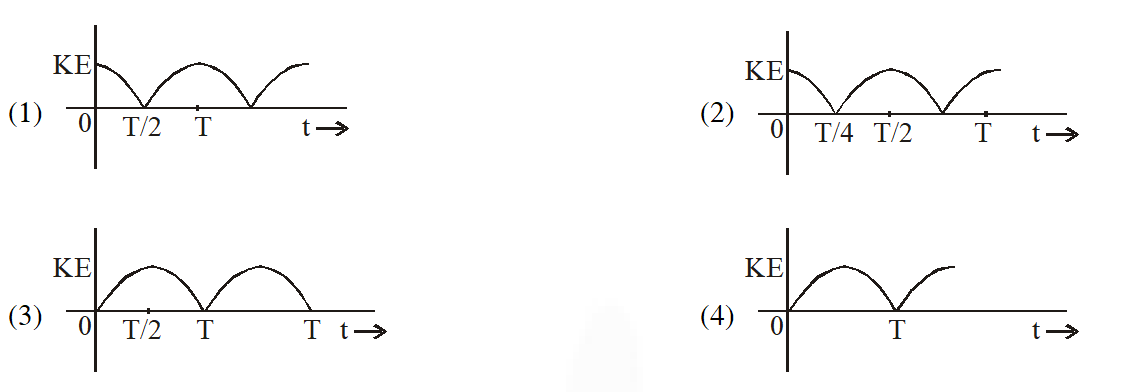[JEE Mains-2017]
Ans. (2)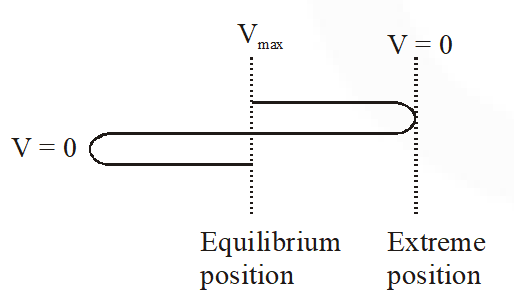Time taken to reach the extreme position from equilibrium position is $\frac{\mathrm{T}}{4}$. Velocity is maximum at equilibrium position and zero at extreme position. $\mathrm{V}=\mathrm{A} \omega \cos \omega \mathrm{t}$ $\mathrm{K.E.}=\frac{1}{2} \mathrm{mv}^{2}$ (m is the mass of particle and v is the velocity of particle $\mathrm{K.E.}=\frac{1}{2} \mathrm{mA}^{2} \omega^{2} \cos ^{2} \omega \mathrm{t}$ Hence graph of K.E. v/s time is square cos function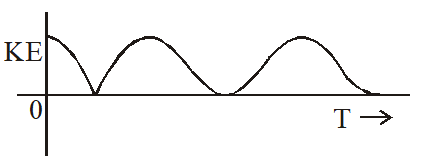Q. frequency of $10^{12} / \mathrm{sec} .$ What is the force constant of the bonds connecting one atom with the other ? (Mole wt. of silver $=108$ and Avagadro number $=6.02 \times 10^{23} \mathrm{gm}$ mole $^{-1}$ ) (1) 7.1 N/m                (2) 2.2 N/m                 (3) 5.5 N/m              (4) 6.4 N/m [JEE Mains-2018]
Ans. (1) Time period of SHM is given by $\mathrm{T}=2 \pi \sqrt{\frac{\mathrm{m}}{\mathrm{k}}}$ frequency $=\frac{1}{2 \pi} \sqrt{\frac{\mathrm{k}}{\mathrm{m}}}=10^{12}$ where m = mass of one atom $=\frac{108}{\left(6.02 \times 10^{23}\right)} \times 10^{-3} \mathrm{kg}$ $\frac{1}{2 \pi} \sqrt{\frac{\mathrm{k}}{108 \times 10^{-3}} \times 6.02 \times 10^{23}}=10^{12}$ On solving K = 7.1 N/m

Aryan from Allen phone no hai 73096 01741
July 16, 2021, 12:38 p.m.
Bekar hai sala demotivate kr deta hai
hacker
May 15, 2021, 2:37 p.m.
what is formula of maximum velocity
Prani
May 15, 2021, 2:35 p.m.
Excellent.....thank you so much...nice app
TANU
March 1, 2021, 6:04 p.m.
VERY EFFECTIVE
Jan. 19, 2021, 5:51 p.m.
Site is not working properly
Avinash
Dec. 22, 2020, 8 p.m.
Excellent
eSARAL
July 21, 2020, 9:39 a.m.
10% for 100%
eSARAL
July 21, 2020, 9:38 a.m.